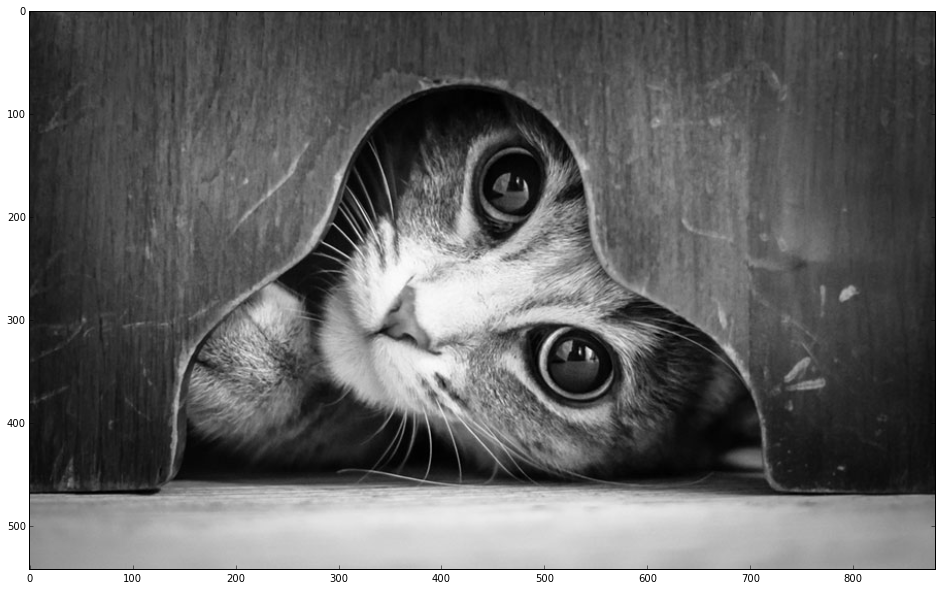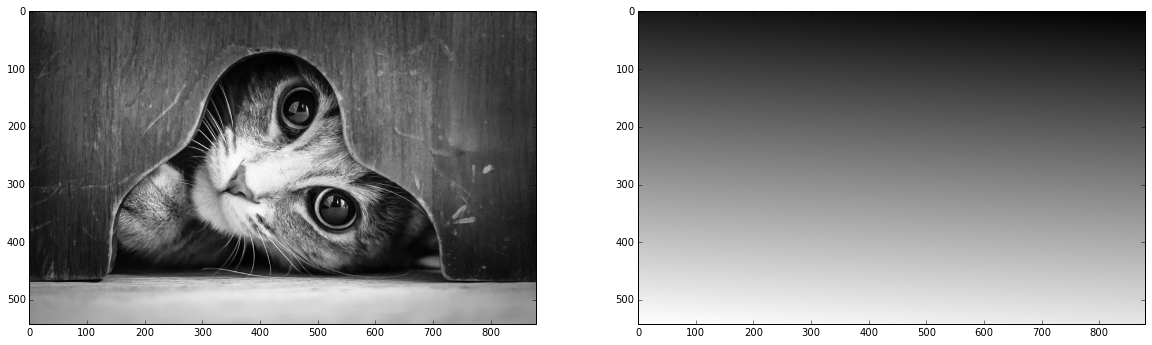# Reconstructing pictures with machine learning [demonstration]¶

In this post I demonstrate how different techniques of machine learning are working.

The idea is very simple:

• each black & white image can be treated as a function of 2 variables - x1 and x2, position of a pixel
• intensity of a pixel is output
• this 2-dimentional function is very complex
• we can leave only a small fraction of pixels, treating others as 'lost'
• by looking how different regression algorithms reconstruct the picture, we can get some understanding of how these algorithms are operating

Don't treat this demonstration as some 'comparison of approaches', because this problem (reconstructing a picture) is very specific and has very few in common with typical ML datasets and problems. And of course, this approach is not to be used in practice to reconstruct pictures :)

I am using scikit-learn and making use of its API, enabling user to construct new models via meta-ensembling and pipelines.

## First, we import lots of things¶

In :
# !pip install image
from PIL import Image
%pylab inline

Populating the interactive namespace from numpy and matplotlib

In :
import numpy
from sklearn.pipeline import make_pipeline

from sklearn.cross_validation import train_test_split
from sklearn.random_projection import GaussianRandomProjection
from sklearn.tree import DecisionTreeRegressor
from sklearn.linear_model import LinearRegression
from sklearn.kernel_approximation import RBFSampler
from sklearn.preprocessing import StandardScaler
from sklearn.svm import SVR
from sklearn.neighbors import KNeighborsRegressor

from rep.metaml import FoldingRegressor
from rep.estimators import XGBoostRegressor, TheanetsRegressor


I took quite complex picture with many little details

In :
!wget http://static.boredpanda.com/blog/wp-content/uploads/2014/08/cat-looking-at-you-black-and-white-photography-1.jpg  -O image.jpg

--2016-02-16 15:40:20--  http://static.boredpanda.com/blog/wp-content/uploads/2014/08/cat-looking-at-you-black-and-white-photography-1.jpg
Resolving static.boredpanda.com... 94.31.29.99
Connecting to static.boredpanda.com|94.31.29.99|:80... connected.
HTTP request sent, awaiting response... 200 OK
Length: 80728 (79K) [image/jpeg]
Saving to: 'image.jpg'

image.jpg           100%[=====================>]  78.84K  --.-KB/s   in 0.05s

2016-02-16 15:40:20 (1.41 MB/s) - 'image.jpg' saved [80728/80728]


In :
image = numpy.asarray(Image.open('./image.jpg')).mean(axis=2)

plt.figure(figsize=[20, 10])
plt.imshow(image, cmap='gray')

Out:
<matplotlib.image.AxesImage at 0x1184b6150>## Define a function to train regressor¶

train_size is how many pixels shall be used in reconstructing the picture. By default, the algorithm will use only 2% of pixels

In :
def train_display(regressor, image, train_size=0.02):
height, width = image.shape
flat_image = image.reshape(-1)
xs = numpy.arange(len(flat_image)) % width
ys = numpy.arange(len(flat_image)) // width
data = numpy.array([xs, ys]).T
target = flat_image
trainX, testX, trainY, testY = train_test_split(data, target, train_size=train_size, random_state=42)
mean = trainY.mean()
regressor.fit(trainX, trainY - mean)
new_flat_picture = regressor.predict(data) + mean
plt.figure(figsize=[20, 10])
plt.subplot(121)
plt.imshow(image, cmap='gray')
plt.subplot(122)
plt.imshow(new_flat_picture.reshape(height, width), cmap='gray')


## Linear regression¶

not very surprising result

In :
train_display(LinearRegression(), image)## Decision tree limited by depth¶

In :
train_display(DecisionTreeRegressor(max_depth=10), image)